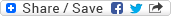## Wednesday, May 21, 2008

### What is the age of the oldest child who plays Piano?

Puzzle: Two freinds (say A & B) see each other after a very long time, say 20 years. After a brief conversation, A finds that B is married and having 3 children. When inquired about the age of the children B replies to A "The product of their ages is 72 and the sum of their ages is same as the number on that building (pointing to a building)". A tries to think for a while and then says "Oh... I couldn't find the ages". Then B promptly realizes something and responds "The oldest plays Piono". Now A smiles and says that his oldest child is also of the same age. How old is the oldest child of A (or B)? Consider that all the ages are natural numbers only.

Solution: The only concrete piece of info we have here is that the product of three natural numbers is 72. We can think of all such triplets in not more than few minutes. But, this info is not sufficient. A can see the number on the bulding that means he know the sum and still couldn't find the triplet. Therefore, there would be more than one triplet for the same sum. Otherwise A would have easily found the correct triplet and in turn the age of the children. Right?

B's reponse brings up only one point that the highest of the correct triplet is unique and then only he can say that the oldest child plays piano.

Good. We have enough info now. We just need to write down the triplets now and the answer will be quite apparent then. The triplets satisfying the first info (product being 72) are: (1, 1, 72 -> Sum = 74), (1, 2, 36 -> Sum = 39), (1, 3, 24 -> Sum = 28), (1, 4, 18 -> Sum = 23), (1, 6, 12 -> Sum = 19), (1, 8, 9 -> Sum = 18), (2, 2, 18 -> Sum = 22), (2, 3, 12 -> Sum = 17), (2, 4, 9 -> Sum = 15), (2, 6, 6 -> Sum = 14), (3, 3, 8 -> Sum = 14), and (3, 4, 6 -> Sum = 13).

You can easily see that there are two triplets with the same 'sum'. Hence, the building next to A & B has this number '14'. These triplets are (2, 6, 6) and (3, 3, 8).

Now, the info 'the oldest' eliminates the triplet (2, 6, 6) as in this case the highest number has two occurrences and hence A would not be having a single 'oldest child'. Instead he would have been having twins in that case :-)

So, the only choice left is (3, 3, 8) and hence the oldest child of A (or B as both are of the same age) is 8 years old.